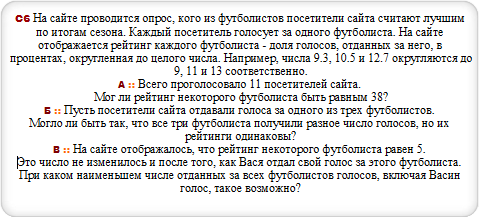# Закажи себе WolframAlpha|Pro! Получи пошаговые решения твоих задач!

## Реальный ЕГЭ :: 05.06.2014 :: Восток 1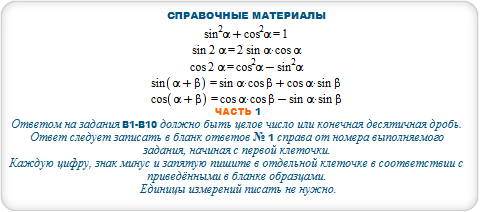#### R = m*s-S where S=450, s=24, m=25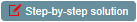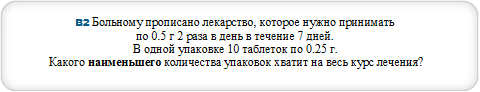#### ceil ((k*p*r)/(m*s)) where k=7, m=10, p=2, r=0.4, s=0.25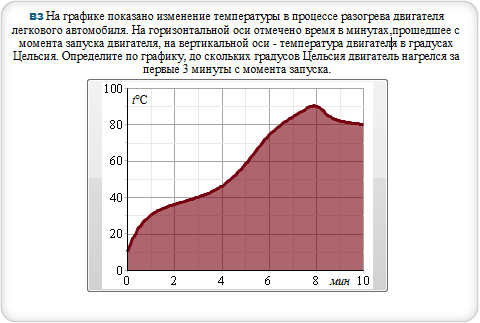#### {{0,10},{0.5,23},{1,30},{2,36},{3,40},{4,46},{5,58},{6,74},{7,84},{7.5,88},{8,90},{8.5,85},{9,82},{10,80}}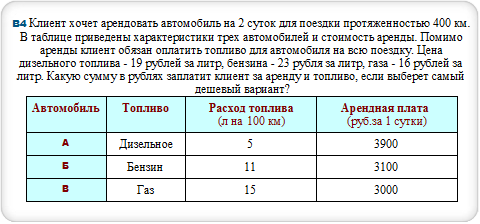#### M*P+(S*c*p)/100 where M=2, P=3000, S=400, p=15, c=16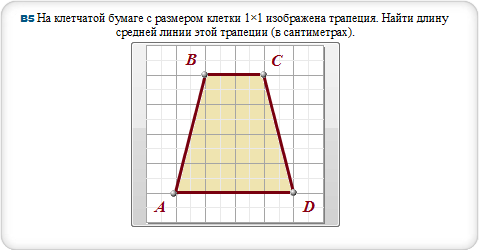#### polygon (0,0),(1,4),(3,4),(4,0)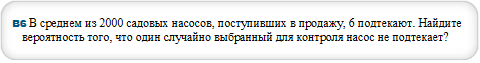#### p=N((n-m)/n) for m = 6, n = 2000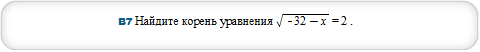#### sqrt(-32-x) = 2 over the reals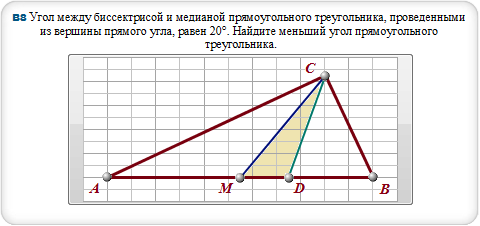#### polygon (0,0),(9.06, 4.22),(5.51, 0),(7.52, 0),(9.06, 4.22),(11.03, 0),(0,0)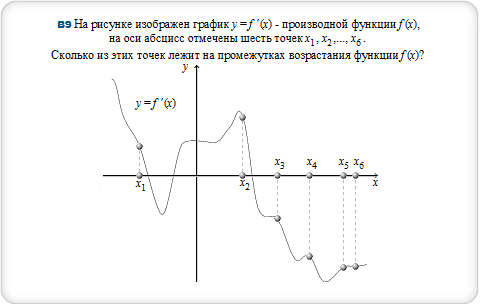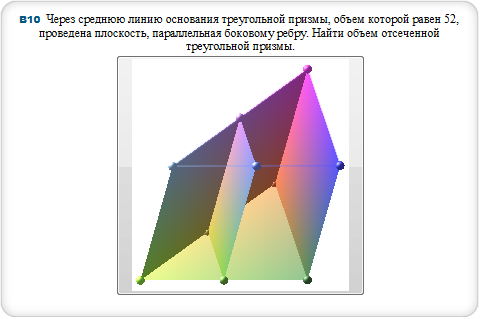#### triangular prism with edge length 1 and triangular prism with edge length 2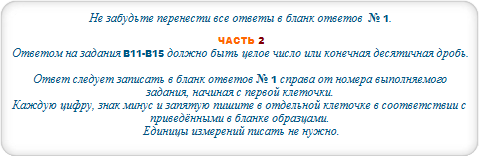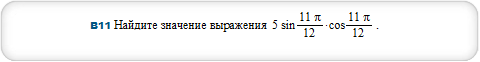#### simplify 5*sin((11*Pi)/12)*cos((11*Pi)/12)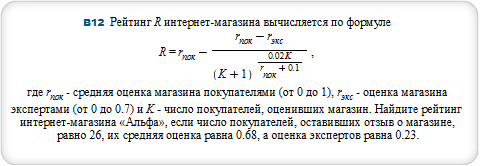#### R = p-(p-r)/(K+1)^((0.02*K)/(p+.1)) where p=.68,r=.23,K=26#### cone with height 10 and radius 6#### x = w*((m+n-2*p)/(m-n)) where m=10,n=35,p=30,w=225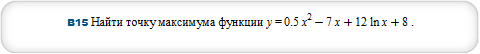#### local extrema x^2/2-7*x+12*ln(x)+8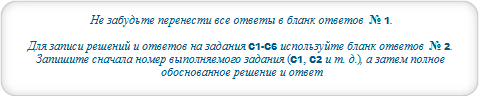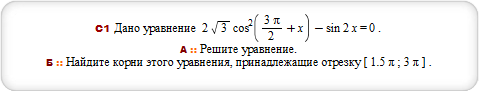#### plot {2*sqrt(3)*cos(3*Pi/2+x)^2,sin(2*x)} for x=3*Pi/2..3*Pi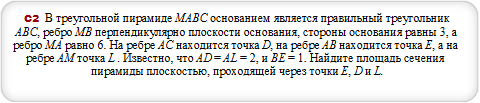#### plot (36^(x-1/2)-7*6^(x-1)+1, x*log(4,-x^2-3*x+5)) for x=-5..2, y=-4..2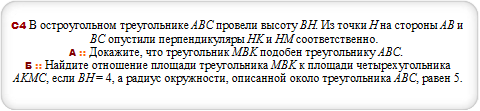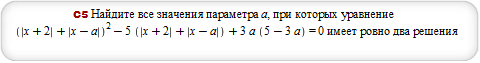#### solve |x+2|+|x-a| = 5-3*a over the reals